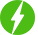# Graphical Representation of Linear Equations Part-I

1

Graphical Representation of Linear Equations Part-I, Linear Equations in two variables.

## Course Description

1. How to geometrically represent a linear equation in one variable?

2. How can we geometrically represent a linear equation in two variables when only one variable is given in the question?

3. How to draw the graph of linear equation in two variables?

4. How to write the equations of two lines passing through a solution given in the question? How many more such lines are there, and why?

5. If a point (i.e. values of x and y given) lies on the graph of a linear equation in two variables, then how to find the value of coefficient of one variable given in the question?

6. The taxi fare in a city is given in the question where fare for first km. is given and the rate of fare for subsequent distance is given per km. Distance covered and total fare are given in the question as x km. and Rs.y respectively. How to write a linear equation for the above information and also to draw its graph.

7. What is the meaning of Linear equation in two variables?

8. What is the standard form of Linear Equation in two variables?

9. What is the highest power of x and y in the Linear equation?

10. What is the shape formed when we graphically represent a linear equation in two variables?

Free \$74.99
We will be happy to hear your thoughtsRegister New Account
• Total (0)
0